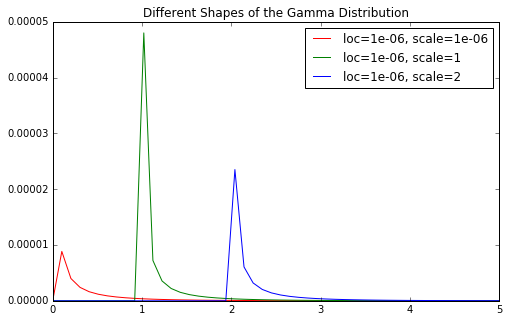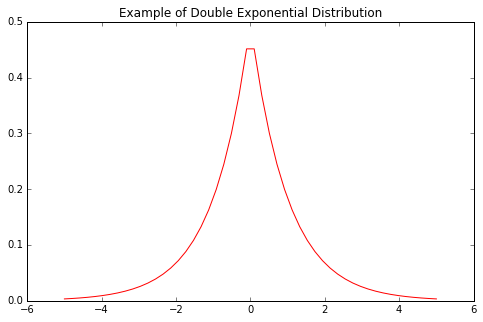# 贝叶斯岭回归

## 准备模拟数据

 ``````1 2 `````` ``````from sklearn.datasets import make_regression X, y = make_regression(1000, 10, n_informative=2, noise=20) ``````

## sklearn 调用bayesian ridge

 ``````1 2 `````` ``````from sklearn.linear_model import BayesianRidge br = BayesianRidge() ``````

α超参数，`lambda_*`是先验概率分布的λ

λ超参数。

 ``````1 2 `````` ``````br.fit(X, y) br.coef_ ``````
 ``````1 2 3 4 `````` ``````array([ 1.61121595e-01, 6.14013495e-01, -9.47984731e-02, 1.21647463e+00, -3.56269283e-01, -8.25356834e-02, -8.79509253e-01, 8.68182936e+01, 3.77686282e+01, -7.82795526e-01]) ``````

 ``````1 2 3 `````` ``````br_alphas = BayesianRidge(alpha_1=10, lambda_1=10) br_alphas.fit(X, y) br_alphas.coef_ ``````
 ``````1 2 3 4 `````` ``````array([ 1.60231407e-01, 6.12504197e-01, -9.21040743e-02, 1.21254421e+00, -3.58470294e-01, -8.59685982e-02, -8.80643964e-01, 8.67400560e+01, 3.77362149e+01, -7.81155501e-01]) ``````

## 原理

α参数，先验概率分布都服从Γ

Γ分布。

Γ

Γ分布是一种极具灵活性的分布。不同的形状参数和尺度参数的Γ

Γ分布形状有差异。1e-06是 scikit-learn里面`BayesianRidge`形状参数的默认参数值。

 `````` 1 2 3 4 5 6 7 8 9 10 11 12 13 14 15 16 17 18 `````` ``````import matplotlib.pyplot as plt %matplotlib inline from scipy.stats import gamma import numpy as np form = lambda x, y: "loc={}, scale={}".format(x, y) g = lambda x, y=1e-06, z=1e-06: gamma.pdf(x, y, z) g2 = lambda x, y=1e-06, z=1: gamma.pdf(x, y, z) g3 = lambda x, y=1e-06, z=2: gamma.pdf(x, y, z) rng = np.linspace(0, 5) f, ax = plt.subplots(figsize=(8, 5)) ax.plot(rng, list(map(g, rng)), label=form(1e-06, 1e-06), color='r') ax.plot(rng, list(map(g2, rng)), label=form(1e-06, 1), color='g') ax.plot(rng, list(map(g3, rng)), label=form(1e-06, 2), color='b') ax.set_title("Different Shapes of the Gamma Distribution") ax.legend(); ``````### 进一步理解

 ``````1 2 3 4 5 6 7 8 `````` ``````from scipy.stats import laplace form = lambda x, y: "loc={}, scale={}".format(x, y) g = lambda x: laplace.pdf(x) rng = np.linspace(-5, 5) f, ax = plt.subplots(figsize=(8, 5)) ax.plot(rng, list(map(g, rng)), color='r') ax.set_title("Example of Double Exponential Distribution"); ``````